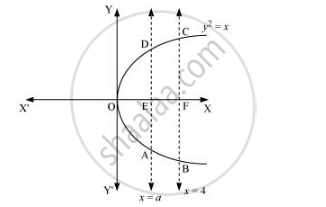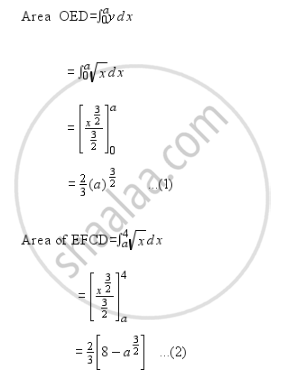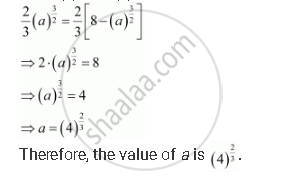Share

Books Shortlist

# The Area Between X = Y2 And X = 4 is Divided into Two Equal Parts by the Line X = A, Find the Value Of A. - CBSE (Science) Class 12 - Mathematics

#### Question

The area between x = y2 and x = 4 is divided into two equal parts by the line x = a, find the value of a.

#### Solution

The line, x = a, divides the area bounded by the parabola and x = 4 into two equal parts.

∴ Area OAD = Area ABCDIt can be observed that the given area is symmetrical about x-axis.

⇒ Area OED = Area EFCDFrom (1) and (2), we obtainIs there an error in this question or solution?

#### APPEARS IN

NCERT Solution for Mathematics Textbook for Class 12 (2018 to Current)
Chapter 8: Application of Integrals
Q: 8 | Page no. 366
Solution The Area Between X = Y2 And X = 4 is Divided into Two Equal Parts by the Line X = A, Find the Value Of A. Concept: Area Under Simple Curves.
S